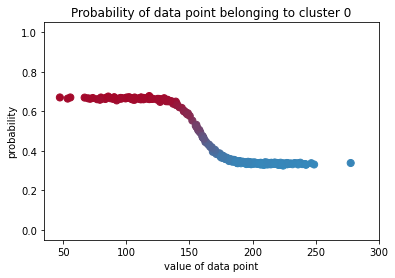# Trying to reproduce the gaussian mixture example from bayesian-methods-for-hackers with numpyro

Hi everyone! I’m starting exploring this package and I had some problems recreating the gaussian mixture example from bayesian methods for hackers (https://github.com/CamDavidsonPilon/Probabilistic-Programming-and-Bayesian-Methods-for-Hackers/blob/master/Chapter3_MCMC/Ch3_IntroMCMC_PyMC3.ipynb).

This is my snippet

``````from jax import random
import jax.numpy as jnp
import numpyro
import numpyro.distributions as dist
from numpyro.infer import MCMC, NUTS
import pandas as pd

def model(data):
with numpyro.plate('samples', len(data)):
p_i = numpyro.sample('p_i', dist.Uniform(0, 1))
c_i = numpyro.sample('c_i', dist.Bernoulli(p_i))

mus = numpyro.sample('mus', dist.Normal(jnp.array([120, 190]), 10).to_event())
sds = numpyro.sample('sds', dist.Uniform(0, 100).expand().to_event())

center_i = mus[c_i]
sd_i = sds[c_i]

with numpyro.plate('samples', len(data)):
obs = numpyro.sample('obs', dist.Normal(center_i, sd_i), obs=data)

data = data.values.flatten()

rng_key = random.PRNGKey(42)
rng_key, rng_key_ = random.split(rng_key)

kernel = NUTS(model)

num_samples = 2000
mcmc = MCMC(kernel, num_warmup=1000, num_samples=num_samples)

mcmc.run(
rng_key_, data=data
)
``````

The problem is that in the example linked above, the averages of the `p_i` ranges from `0` to `1` as reported in the following plot

while in my implementation using `numpyro` they range only between about `0.35` and `0.65`:Is it related to some problem/bug with my implementation or could be some convergence issue?

Thanks!

@salvomcl I think you might try to use global `p` as in the original article and infer_discrete or DiscreteHMCGibbs.